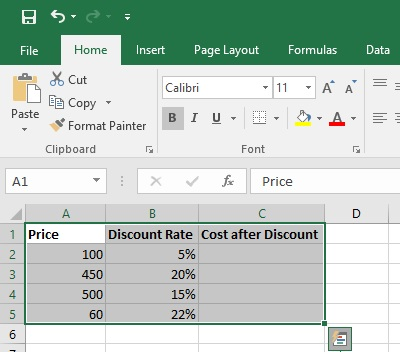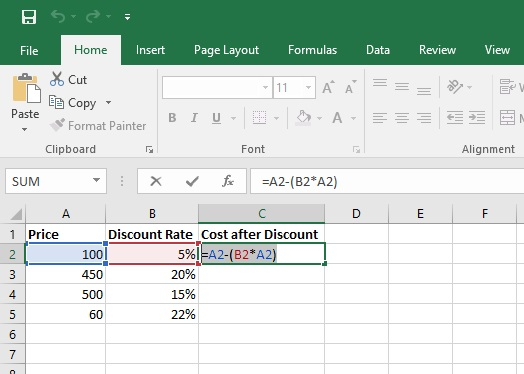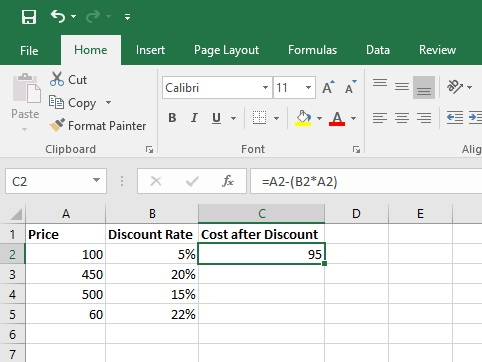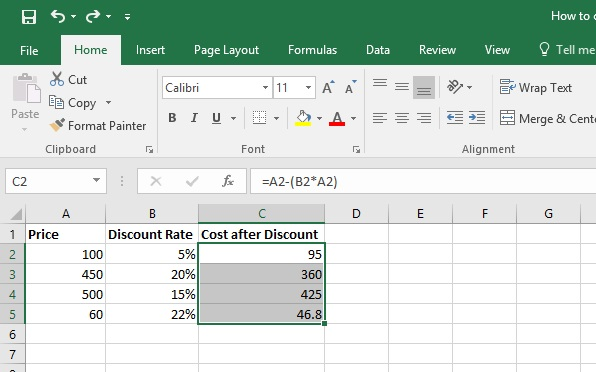[email protected] +1 203-349-9909 / +91-8080042523

# How to Calculate a Percentage Increase in Excel

Percentage increase calculator

Sometimes percentages can be frustrating because it’s not always easy to remember what we learned about them in school. Let Excel do the work for you – simple formulas can help you find the percentage of a total and total cost after the discount.

Let see how excel can help us to find out the cost of the product after discount.

## Let’s calculate percentage increase in Excel

Imagine the problem we’re trying to solve. We want to know the total cost after the discount. A discount is a percentage of the original price.

To make this problem easier to solve with a spreadsheet, we’ll break it down into two steps:

1. Find the discount
2. Subtract that value from the original total to find the final cost.

Let’s go through the following steps:Steps 1: Create a table in excel sheet same as like given picture. Column A is showing the Price list, Column B is for a Discount rate as a percentage (%) and column C is for Discount price or Cost after discount.

If you have lists of data about the original prices and discount rate in a worksheet, you can calculate the sales prices after discount.Step 2: In Cell C2 Enter the formula given below:

=A2-(B2*A2)

This formula is telling to Excel that multiply the discount rate B2 with actual price A2 then subtract the result from actual price A2.Step 3: Now press ENTER and see the result. In this example Actual price was 100 and discount rate was 5%, So normally we can easily calculate that the discount price would be obviously 95. So, it proves the accuracy of our formula.Step 4: Use the same formula for another price. So input the formula in another cells of column C in the same way, then press ENTER. After that our table with discount prices result will be looked like above picture.

#### Tips: If you change the actual price or discount rate of any cell, then the result of discount price will be changed automatically. In this way you can use this again if your price or discount change in future.

Now we have successfully learned how to calculate from percentage to discount price using Excel….!!